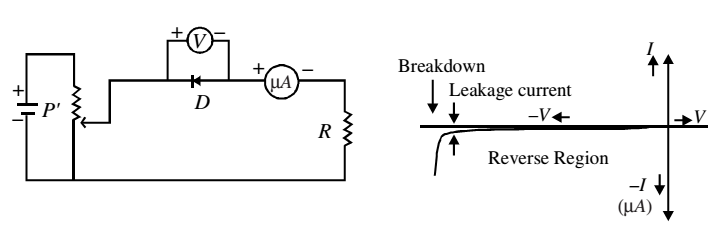# Characteristics of p-n Junction Diodes

The practical application of a semiconductor device in electronic circuits depends on the current and voltage (I-V) relationship, as it gives vital information to a circuit designer as well as a technician. Therefore, with the help of I–V characteristics, you can know how much current flows through the junction diode at a particular voltage.

### Forward Bias Characteristics

To draw forward bias characterstic of a p-n junction diode, the positive terminal of a battery (B) is connected to p-side of the diode through the rheostat. The voltage applied to the diode can be varied with the help of the rheostat. The milliammeter (mA) measures the current in the circuit and voltmeter (V) measures the voltage across the diode.The direction of conventional current is the same as the direction of the diode arrow. Since current experiences little opposition to its flow through a forward biased diode and it increases rapidly as the voltage is increased, a resistance (R) is added in the circuit to limit the value of current. If this resistance is not included, the diode may get permanently damaged due to flow of excessive current through it.

The characteristic curve does not pass through origin; instead it meets the V-axis around 0.7 V. It means that the p-n junction does not conduct until a definite external voltage is applied to overcome the barrier potential. The forward voltage required to get the junction in conduction mode is called knee voltage. It is about 0.7 V for Si and 0.3 V for Ge p-n junction.

This voltage is needed to start the hole-electron combination process at the junction. As the applied voltage is increased beyond knee voltage, the current through the diode increases linearly. For voltage of around 1 V, the current may attain a value of 30-80 mA.

### Reverse Bias Characteristics

To draw reverse bias characteristics of a p-n junction, the terminals of the junction are reversed and instead of milliammeter, microammeter (µA) is used.The junction current is comparatively much less in reverse bias for all voltages below the breakdown voltage. And at breakdown voltage, the current increases rapidly for a small increase in voltage.

A p-n junction diode offers low resistance when it is forward biased and high resistance when reverse biased. At the breakdown voltage in reverse biased p-n junction diode, the sharp increase in reverse current is due to sudden decrease in resistance offered by the junction.

Thus, a p-n junction diode conducts in only one direction, i.e. has unidirectional conduction of current, with electrons flowing from the n-type region to p-type end in forward bias.